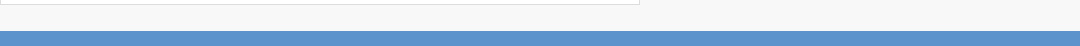# Internal Rate of Return

The Internal Rate of Return (IRR) is a much referenced metric yet, often misunderstood concept. The IRR measures the profitability of potential investments. It is the discount rate that converts the net present value (NPV) of all cash flows from a particular project to equal zero. The formula involves the net cash inflow, investment cost, number of time period(s) and discount rate(s). However, the IRR cannot be calculated analytically, but only through trial-and-error or a specific software program.

In theory, any project with an IRR quarter than its cost of capital is a profitable one. Usually, in ranking potential expenditures, the highest IRR determines the most economic rate of return. Shorter duration projects may have a high IRR, but may not add large value to the investor (company) over time. Modifications to IRR should be considered for long-term investments, since fluctuating cash flows over that period may best be characterized by multiple, distinct IRR's. These mods would incorporate the cost of capital at the rate at which cash flows are invested.

Why is any of the above relevant to valuations: The Mentor group utilizes IRR calculations as a "sanity check" for our financial valuations – business, intellectual property (IR), derivatives, discounting analysis, allocation of purchase price, and expert testimony. Application of the IRR, along with our Monte Carlo simulation, greatly limits the standard deviation of reasonableness.

Categories: General